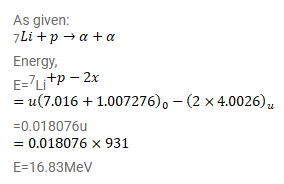# How much energy is released in the following reaction?

Question:

How much energy is released in the following reaction?

$7^{L i}+p \rightarrow \alpha+\alpha$

Atomic mass of $7 \mathrm{Li}=7.0160 \mathrm{u}$ and that of ${ }^{4} \mathrm{He}=4.0026 \mathrm{u}$.

Solution: